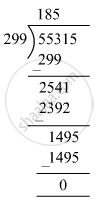# On dividing 55390 by 299, the remainder is 75. Find the quotient using the division algorthm. - Mathematics

Fill in the Blanks

On dividing 55390 by 299, the remainder is 75. Find the quotient using the division algorthm.

#### SolutionHere, Dividend = 55390, Divisor = 299 and Remainder = 75
We have to find the quotient.
Now, Dividend = Divisor × Quotient + Remainder
⇒ 55390 = 299 × Quotient + 75
⇒ 55390 − 75 = 299 × Quotient
⇒ 55315 = 299 × Quotient
⇒ Quotient = 55315 ​÷ 299
Hence, quotient =185

Is there an error in this question or solution?

#### APPEARS IN

RS Aggarwal Class 6 Mathematics
Chapter 3 Whole Numbers
Exercise 3E | Q 7 | Page 56